# Raman Amplifiers

Fiber-based Raman amplifiers make use of stimulated Raman scattering (SRS) occurring in silica fibers. The following figure shows how a fiber can be used as a Raman amplifier in the forward-pumping configuration. The pump and signal beams at frequencies ωp and ωs are injected into the fiber through a fiber coupler. During the SRS process, a pump photon gives up its energy to create another photon of reduced energy at the signal frequency; the remaining energy is absorbed by the silica material in the form of molecular vibrations (optical phonons). The energy is transferred continuously fro the pump to the signal through SRS as the two beams co-propagate inside the fiber. The pump and signal beams counter-propagate in the backward-pumping configuration often used in practice.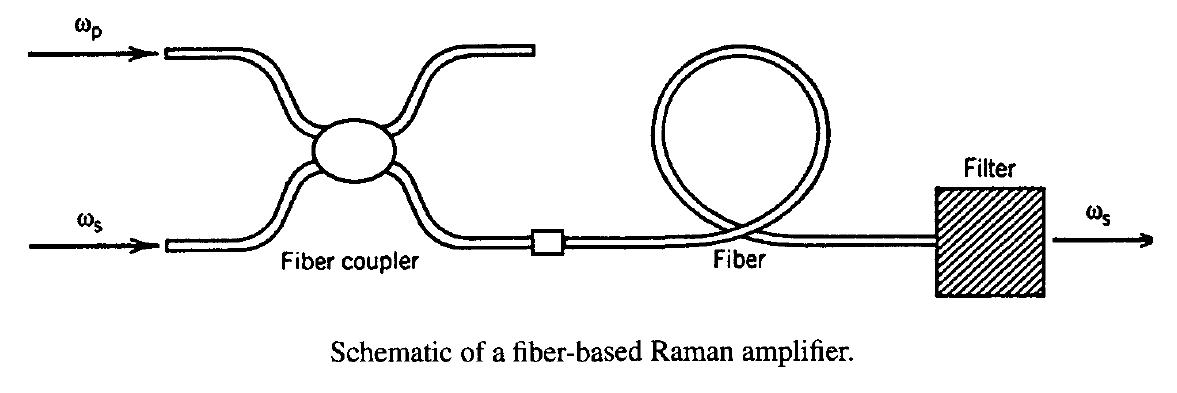#### 1. Raman Gain and Bandwidth

The Raman-gain spectrum of silica fibers is shown in the figure below; its broadband nature is a consequence of the amorphous nature of glass.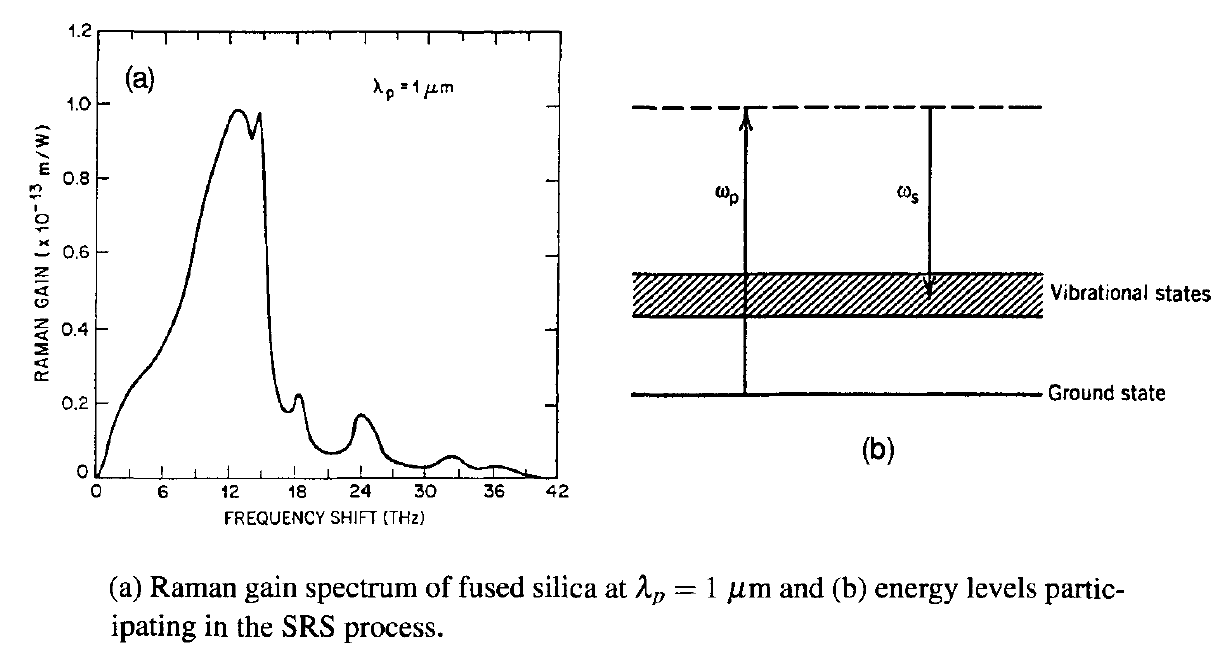The Raman-gain coefficient gR is related to the optical gain g(z) as g = gRIp(z), where Ip is the pump intensity. In terms of the pump power Pp(z), the gain can be written as

g(ω, z) = gR(ω)[Pp(z)/ap]

where ap is the cross-sectional area of the pump beam inside the fiber. Since ap can vary considerably for different types of fibers, the ratio gR/ap is a measure of the Raman-gain efficiency. This ratio is plotted in the figure below for three different fibers.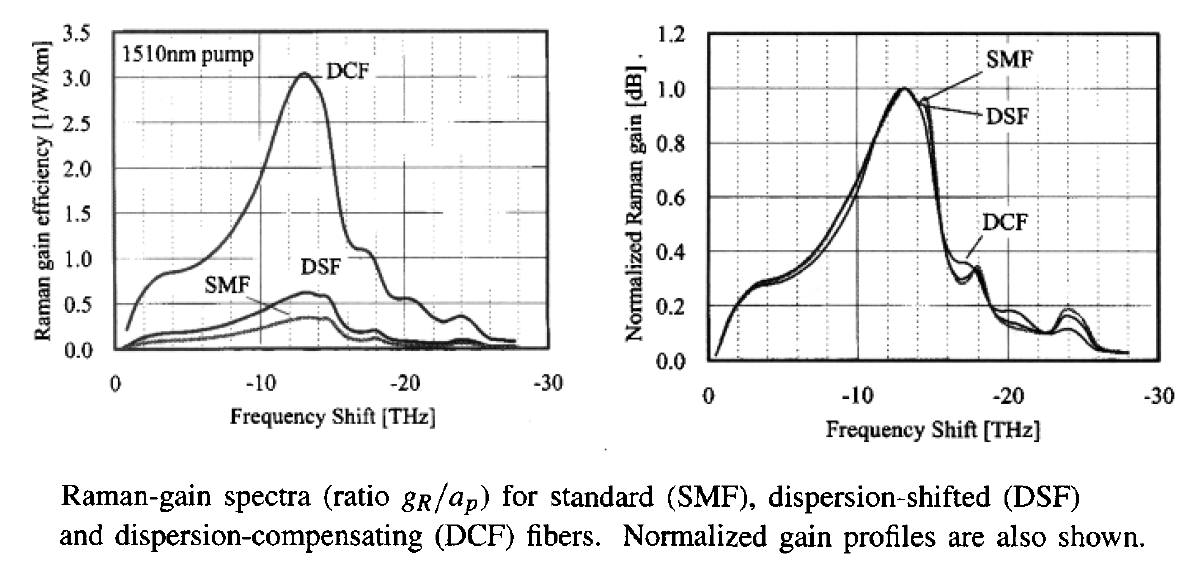A dispersion-compensating fiber (DCF) can be 8 times more efficient than a standard silica fiber (SMF) because of its smaller core diameter. The frequency dependence of the Raman gain is almost the same for the three kinds of fibers as evident from the normalized gain spectra shown in the right side figure above. The gain peaks for a Stokes shift of about 13.2 THz. The gain bandwidth Δνg is nearly 6 THz if we define it as the FWHM of the dominant peak in the figure above.

The large bandwidth of fiber Raman amplifiers makes them attractive for fiber-optic communication systems. However, a relatively large pump power is required to realize an amplification factor G > 20 dB. As an example, if we use G = exp(gL) by ignoring pump losses in the unsaturated region, gL ≈ 6.7 is required for G = 30 dB. By using the measured value of gR = 6 x 10-14 m/W at the gain peak near 1.55 μm with ap = 50 μm2, the required pump power is more than 5 W for a 1-km-long fiber. It can be reduced for longer fibers, but then fiber losses must be included.

Raman amplifiers are called lumped or distributed depending on their design. In the lumped case, a discrete device is made by spooling 1-2 km of a especially prepared fiber that has been doped with Ge or phosphorus for enhancing the Raman gain. The fiber is pumped at a wavelength near 1.45 μm for amplification of 1.55-μm signals. In the case of distributed Raman amplification, the same fiber that is used for signal transmission is also used for signal amplification. The pump light is often injected in the backward direction and provides gain over relatively long lengths (>50 km). The main drawback in both cases from the system standpoint is that high-power lasers are required for pumping. Early experiments often used a tunable color-center laser as a pump; such lasers are too bulky for system applications. For this reason, Raman amplifiers were rarely used during the 1990 when EDFAs became available. The situation changed around 2000 with the availability of compact high-power semiconductor lasers. Since then, both the discrete and distributed Raman amplifiers have been employed in designing lightwave systems.

#### 2. Raman-Induced Signal Gain

Consider the simplest situation in which a single CW pump beam is launched into an optical fiber used to amplify a CW signal. Variations int he pump and signal powers along the amplifier length can be studied by solving two coupled equations. In terms of pump and signal powers, these equations take the form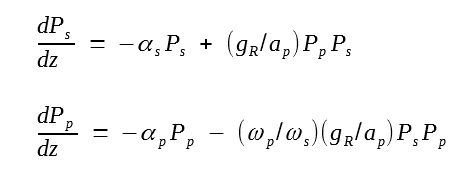where αs and αp represent fiber losses at the signal and pump frequencies, respectively. The frequency ratio ωps results from different energies of pump and signal photons and disappears if these equations are written in terms of photon numbers.

Consider first the case of small-signal amplification for which pump depletion can be neglected (the last term in the second equation above). Substituting Pp(z) = Pp(0)exp(- αpz) in the first equation above, the signal power at the output of an amplifier of length L is given by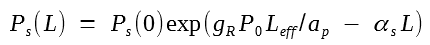where P0 = Pp(0) is the input pump power and Leff = [1 - exp(- αpL)]/αp. Because of fiber losses at the pump wavelength, the effective length of the amplifier is less than the actual length L; Leff ≈ 1/αp for αpL ≫ 1. Since Ps(L) = Ps(0)exp(- αsL) in the absence of Raman amplification, the amplifier gain is given bywhere the small-signal gain g0 is defined as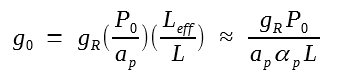The last relation holds for αpL ≫ 1. The amplification factor GA becomes length independent for large values of αpL. The following figure shows variations of GA  with P0 for several values of input signal powers for a 1.3-km-long Raman amplifier operating at 1.064 μm and pumped at 1.017 μm. The amplification factor increases exponentially with P0 initially but then starts to deviate for P0 > 1 W because of gain saturation. Deviations become larger with an increase in Ps(0) as gain saturation sets in earlier along the amplifier length. The solid lines in this figure are obtained by solving equations above numerically to include pump depletion.As the pump supplies energy for signal amplification, it begins to get depleted. A decrease in pump power Pp reduces the optical gain. This reduction in gain is referred to as gain saturation. An approximate expression for the saturated amplifier gain Gs can be obtained assuming αs = αp. The result is given by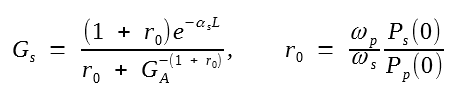The following figure shows the saturation characteristics by plotting Gs/GA as a function of GAr0 for several values of GA.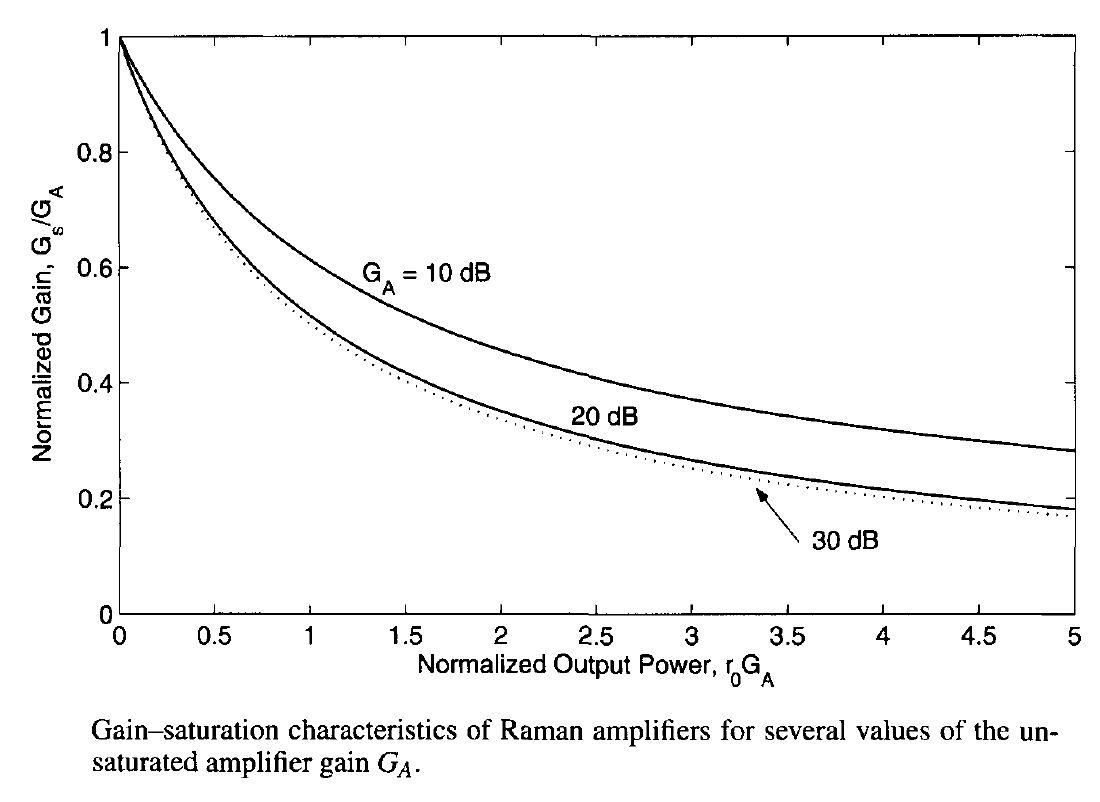The amplifier gain is reduced by 3 dB when GAr0 ≈ 1. This condition is satisfied when the power of the amplified signal becomes comparable to the input pump power P0. In fact, P0 is a good measure of the saturation power. Since typically P0 ~ 1 W, and channel powers in a WDM system are ~1 mW, Raman amplifiers operate in the unsaturated regime, and this following small-signal gain g0 is used in place of Gs.#### 3.  Multiple-Pump Raman Amplification

Starting in 1998, the use of multiple pumps for Raman amplification was pursued for developing broadband optical amplifiers required for WDM lightwave systems operating in the 1.55-μm region. Dense WDM systems (100 or more channels) typically require optical amplifiers capable of providing uniform gain over a 70-80-nm wavelength range. In a simple approach, hybrid amplifiers made by combining erbium doping with Raman gain were used. In one implementation of this idea, nearly 80-nm bandwidth was realized by combining an EDFA with two Raman amplifiers, pumped simultaneously at three different wavelengths (1471, 1495, and 1503 nm) using four pump modules, each module launching more than 150 mW of power into the fiber. The combined gain of 30 dB was nearly uniform over the wavelength range of 1.53-1.61 μm.

Broadband amplification over 80 nm or more can also be realized by using a pure Raman-amplification scheme. In this case, a relatively long span (typically >5 km) of a fiber with a relatively narrow core [such as a dispersion-compensating fiber (DCF)] is pumped using multiple pump lasers. Alternatively, one can use the transmission fiber itself as the Raman-gain medium. In the latter scheme, the entire long-haul fiber link is divided into multiple segments (60 to 100 km long), each one pumped backward using a pump module consisting of multiple pump lasers. The Raman gain accumulated over the entire segment length compensates for fiber losses of that segment in a distributed manner.

The basic idea behind multi-pump Raman amplifiers makes use of the property that the Raman gain exists at any wavelength as long as the pump wavelength is suitably chosen. Thus, even though the gain spectrum for a single pump is not very wide and is flat only over a few nanometers (see figure below), it can be broadened and flattened considerably by using several pumps of different wavelengths.Each pump creates the gain that mimics the spectrum shown in figure (b) above, and superposition of several such spectra can create relatively flat gain over a wide spectral region when pump wavelengths and power levels are chosen judiciously. Figure (a) below shows an example for the case in which five pump lasers operating at wavelengths of 1420, 1435, 1450, 1465, and 1495 nm are used for pumping the Raman amplifier. The individual pump powers are chosen to provide different gain spectra shown in part (b) such that the total Raman gain is nearly uniform over a 80-nm bandwidth (top trace).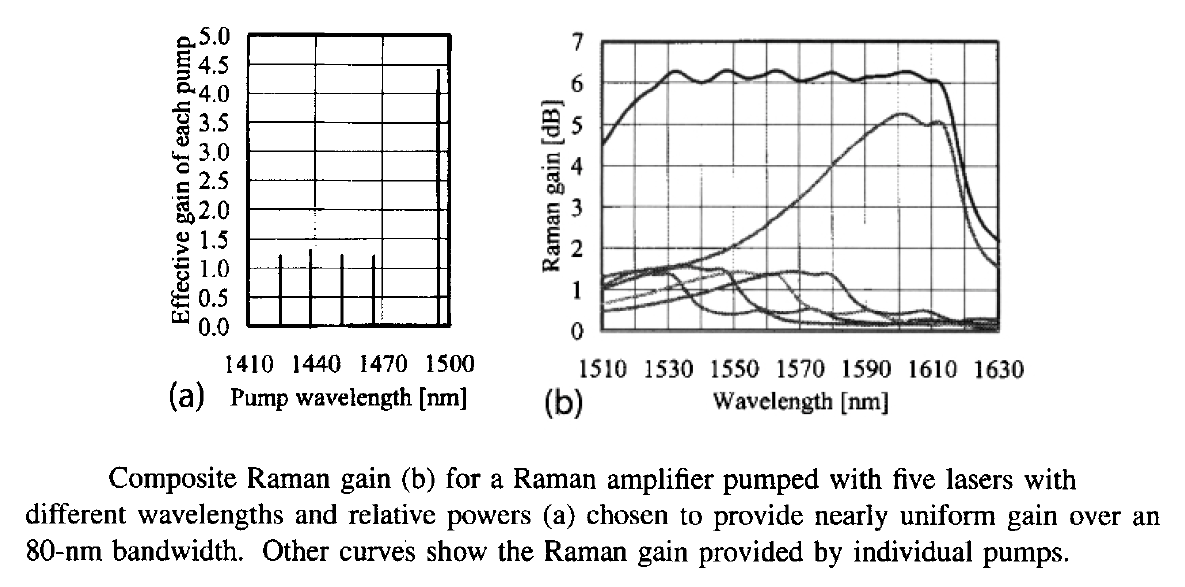Raman gain spectra with a bandwidth of more than 100 nm have been realized using multiple pump lasers. In a 2000 demonstration of this technique, 100 WDM channels with 25-GHz channel spacing, each operating at a bit rate of 10-Gb/s, were transmitted over 320 km. All channels were amplified simultaneously by pumping each 80-km fiber span in the backward direction using four semiconductor lasers. Such a distributed Raman amplifier provided 15-dB gain at a pump power of 450 mW. An undesirable feature of SRS is that the Raman gain is polarization-sensitive. In general, the gain is maximum when the signal and pump are polarized along the same direction but is nearly zero when they are orthogonally polarized. The polarization problem can be solved by pumping a Raman amplifier such that two orthogonally polarized lasers are used at each pump wavelength, or by depolarizing the output of each pump laser.

The design of broadband Raman amplifiers suitable for WDM applications requires consideration of several factors. The most important among them is the inclusion of pump-pump interactions. In general, multiple pump beams are also affected by the Raman gain, and some power from each short-wavelength pump is invariably transferred to each longer-wavelength pump. An appropriate model that includes pump interactions, Rayleigh backscattering, and spontaneous Raman scattering considers each frequency component separately and requires the solution of the following set of coupled equations:where g' = gR/Aeff, μ and ν denote optical frequencies, and the subscripts f and b denote forward- and backward-propagating waves, respectively. A similar equation holds for the backward propagating waves. The parameter nsp accounts for spontaneous Raman scattering that acts as a noise to the amplified signal. It is defined as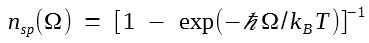where Ω = |μ - ν| is the Raman shift and T denotes absolute temperature of the amplifier. In the first equation above, the first and second terms account for the Raman-induced power transfer into and out of each frequency band. The factor of 4 in the second term results from the polarization-independent nature of spontaneous Raman scattering. Fiber losses and Rayleigh backscattering are included through the last two terms and are governed by the parameters α and rs, respectively.

To design broadband Raman amplifiers, the entire set of such equations is solved numerically to find the channel gains, and input pump powers are adjusted until the gain is nearly the same for all channels. Such amplifiers can amplify 100 channels or more over an 80-nm bandwidth with a ripple of less than 0.1 dB and are suitable for dense WDM systems covering both the C and L bands. Several laboratory experiments have used broadband Raman amplifiers to demonstrate transmission over long distances at high bit rates. In a 2009 experiment, 135 channels, each operating at 111 Gb/s, were transmitted over 6,248 km by using distributed Raman amplification inside a 240-km recirculating fiber loop.

#### 4. Noise Figure of Raman Amplifiers

Spontaneous Raman scattering adds to the amplified signal and appears as a noise because of random phases associated with all spontaneously generated photons. This noise mechanism is similar to the spontaneous emission that affects the performance of EDFAs except that, in the Raman case, it depends on the phonon population in the vibrational state, which in turn depends on temperature of the Raman amplifier.

On a more fundamental level, one should consider the evolution of signal with the noise added by spontaneous Raman scattering by solving the following equation.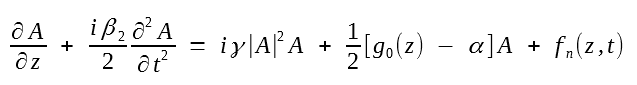If we neglect the dispersive and nonlinear terms in this equation for simplicity, we need to solve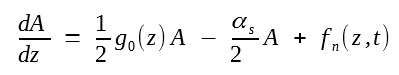where g0(z) = gRPp(z)/ap is the gain coefficient, Pp(z) is the local pump power, and fn(z, t) takes into account the noise added through spontaneous Raman scattering. Since each scattering event is independent of others, this noise can be modeled as a Gaussian stochastic process for which ⟨fn(z, t)⟩ = 0 and the second moment is given by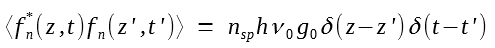with nsp given inThe dA/dz equation above can be easily integrated to obtain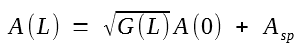where G(L) is the amplification factor and the contribution of spontaneous Raman scattering is given by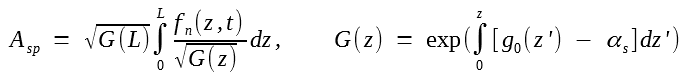It is clear from this equation that the ASE contribution added to the signal depends on the distributed nature of the Raman gain. It is easy to show that an(t) vanishes on average and its second moment is given by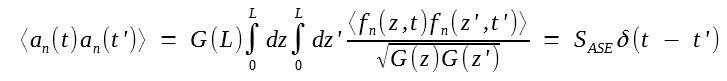where the spectral density of ASE noise is defined as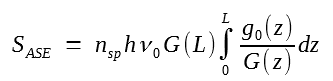We can calculate the power of the ASE contribution using PASE⟨|Asp|2⟩. The result is found to bewhere Δν0 is the bandwidth of the Raman amplifier or of the optical filter, if such a filter is used to reduce the noise. The total noise power is higher by factor of 2 when both polarization components are considered.

The noise figure can now be calculated following the procedure used for EDFAs and is found to be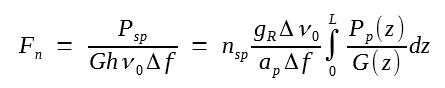This equation shows that the noise figure of a Raman amplifier depends not only on the optical and electrical bandwidths but also on the pumping scheme. It is quite useful for lumped Raman amplifiers for which fiber length is ~1 km and the net signal gain exceeds 10 dB. In the case of distributed amplification, the length of the fiber section typically exceeds 50 km, and pumping is such that G(z) < 1 throughout the fiber length. In this case, Fn predicted by the above equation can be very large. It is common to introduce an effective noise figure using the definition Feff = Fnexp(- αsL). Physically, this concept can be understood by noting that a passive fiber reduces the SNR of an optical signal by a factor of exp(- αsL) because of the reduction in signal power. To find the effective noise figure of a Raman amplifier, one should remove the contribution of the passive fiber.

It should be stressed that Feff can be less than 1 (or negative on the decibel scale). In fact, it is this feature of distributed Raman amplification that makes it so attractive for long-haul WDM lightwave systems. Physically speaking, the distributed gain counteracts fiber losses in the transmission fiber itself and results in a SNR that is improved, compared with the case in which losses are compensated at the end of fiber using lumped amplifiers. The forward pumping results in even less noise because Raman gain is then concentrated toward the input end of the fiber.

The performance of modern Raman amplifiers is affected by several factors that need to be controlled. A few among them are double Rayleigh backscattering, pump-noise transfer, and polarization-mode dispersion. The last one has its origin in the polarization dependence of the Raman-gain coefficient gR in silica fibers. Its impact can be reduced in practice by employing the technique of polarization scrambling. In this technique, the pump polarization is changed randomly so that the signal experiences different local gain in different parts of the fiber, resulting effectively in an average gain that is independent of the signal polarization. The use of spun fibers for Raman amplifiers can also reduce the polarization impairments.